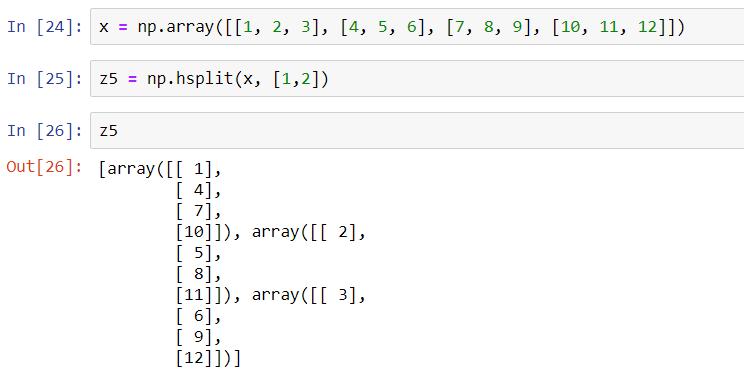Array concatenation means combining two or more arrays into a single array. And array splitting means to split a single array into multiple arrays.

Let us understand, how this is done in NumPy. We will first import the required libraries.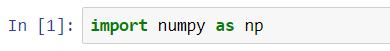### Concatenation of Arrays

For concatenating or joining two or more arrays in NumPy, we have the following functions -

#### np.concatenate([x, y,……z])

This function can concatenate two or more arrays into a single array. By default, the concatenation is done along the 1st axis.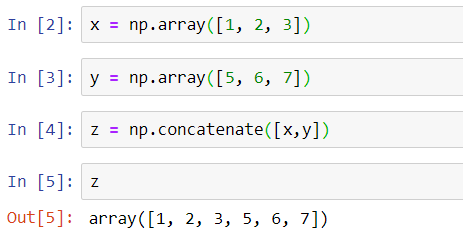We can also concatenate along the 2nd axis or any other axis by explicitly mentioning it.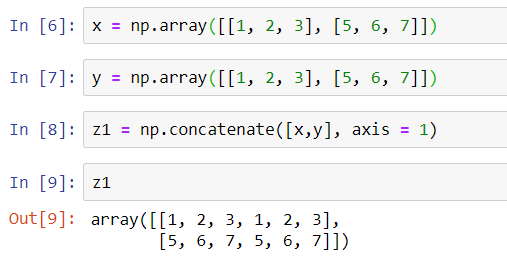#### np.vstack([x,y,…..z])

This function stacks all the arrays vertically.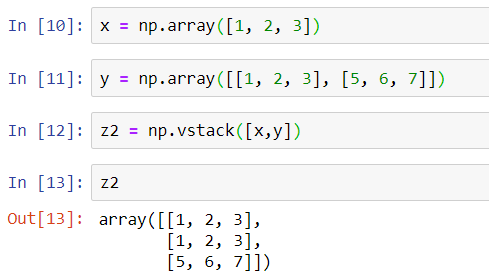#### np.hstack([x,y,….z])

This function stacks all the arrays horizontally.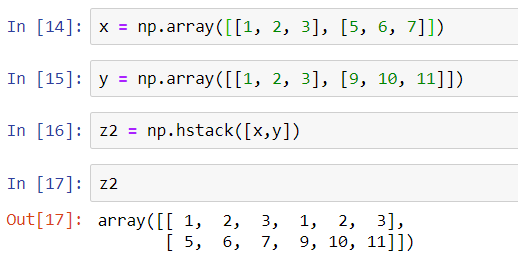### Splitting of Arrays

Splitting is exactly opposite to the concatenation of arrays. For splitting an array in NumPy, we have the following functions -

#### np.split([x], [i1, i2,….in])

This function splits a single array into multiple arrays. The array is split at given indices i1, i2,….in.

In the below given example, we split an array at indices 3 & 5.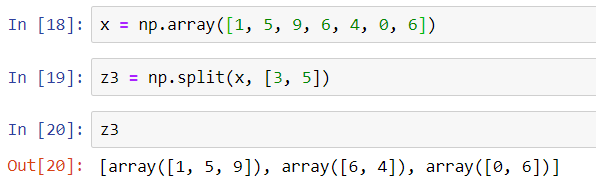#### np.vsplit([x], [i1, i2,….in])

This function splits a single array vertically into multiple arrays at indices i1, i2,….in.

In the below given example, we split an array vertically at indices 2 & 3.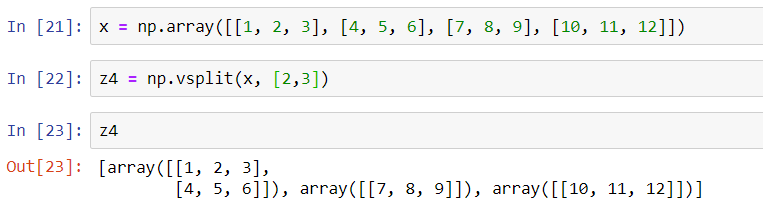#### np.hsplit([x], [i1, i2,….in])

This function splits a single array horizontally into multiple arrays at indices i1, i2,….in.

In the below given example, we split an array horizontally at indices 1 & 2.